# OpenCV单目视觉定位（测量）系统【转载】

2019/7/22 10:07:57 人评论 次浏览 分类：学习教程

OpenCV单目视觉定位（测量）系统

The System of Vision Location with Signal Camera

Abstract:This passage mainly describes how to locate with signalcamera,which bases on OpenCV library.

Key words: OpenCV; Locate;Signalcamera

# 2     预处理

图一 灰度图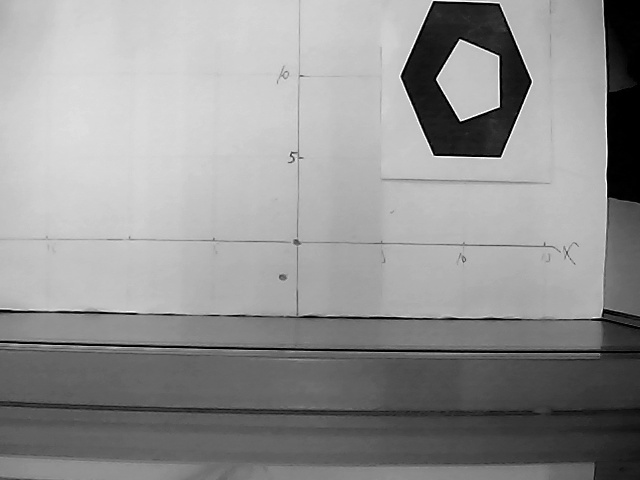# 3.1目标物体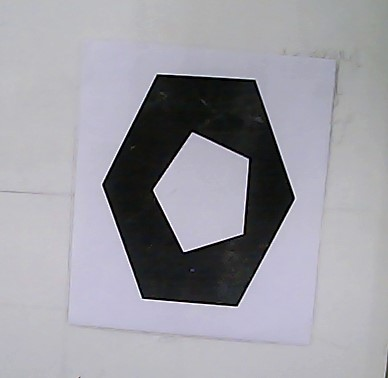图三打印的目标物体

# 3.2 Canny边缘检测

l  低错误性：标识出尽可能多的实际边缘，同时尽可能地减少噪声产生的误报。

l  高定位性：标识出的边缘要与图像中的实际边缘尽可能接近

l  最小效应：图像中的边缘只能标识一次，并且可能存在的图像噪声不应标识为边缘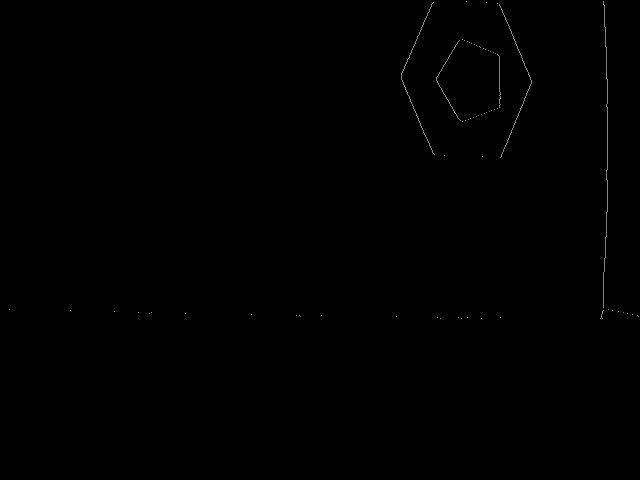图四 边缘检测图

# 3.3 轮廓分析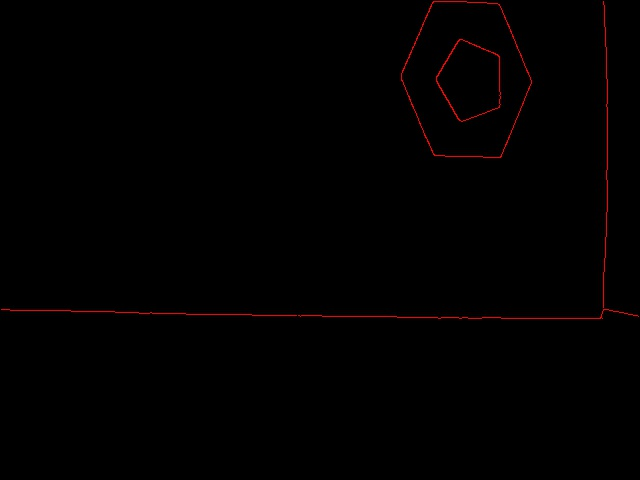# 3       定位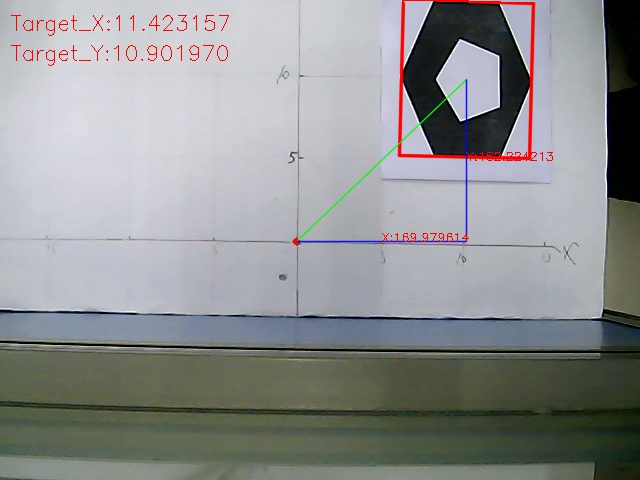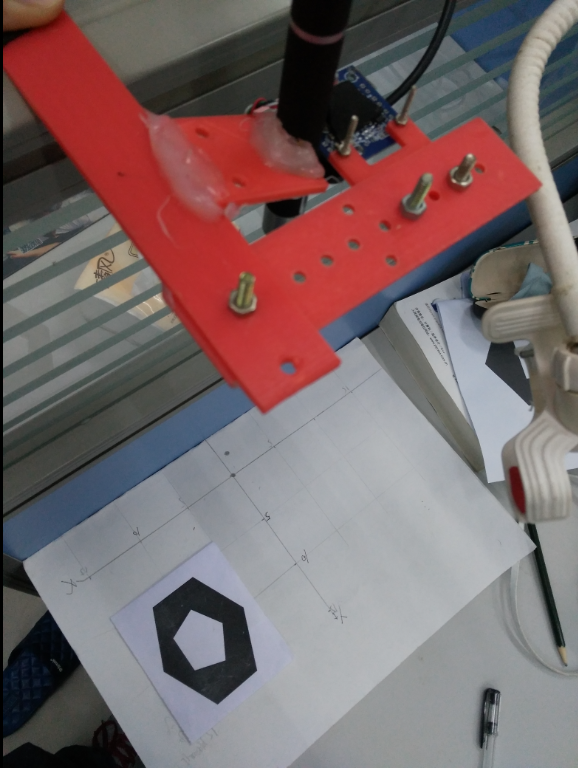图、实验情况

# 核心代码：

`//定位函数void Location(){ 	//均值滤波	blur(gray, gray_blur, Size(3, 3)); 	//边缘检测提取边缘信息	Canny(gray_blur, dstThreshold, 150, 450);	imshow("canny边缘检测", dstThreshold);		//对边缘图像提取轮廓信息    vector<vector<Point> >contours;	findContours(dstThreshold, contours, CV_RETR_LIST, CV_CHAIN_APPROX_SIMPLE);		//画出轮廓	drawContours(contours_image, contours, -1, Scalar(0, 0, 255));	imshow("contours", contours_image); 	//画出定义的原点	circle(src, Point2f(oriX, oriY), 2, Scalar(0, 0, 255), 3); 	//定义分别逼近六边形和五边形的轮廓	vector< vector<Point> > Contour1_Ok, Contour2_Ok; 	//轮廓分析	vector<Point> approx;	for (int i = 0; i < contours.size(); i++){		approxPolyDP(Mat(contours[i]), approx, cv::arcLength(cv::Mat(contours[i]), true)*0.04, true); 		//去除 小轮廓，只提取凸轮廓		if (std::fabs(cv::contourArea(contours[i])) < 600 || !cv::isContourConvex(approx))			continue; 	    //保存逼近六边形的轮廓 到 Contour1_Ok		 if (approx.size() == 6){			Contour1_Ok.push_back(contours[i]);		}	    //保存逼近五边形的轮廓 到 Contour2_Ok		 else if (approx.size() == 5){			 Contour2_Ok.push_back(contours[i]);		} 	} 	//对所有符合要求的六边形，五边形轮廓进行分析	//识别出自定义的物体的关键是：	//1.六边形和五边形轮廓的最小外接矩形的中心基本在同一点	//2.六边形轮廓的最小外接矩形的任一边长大于五边形轮廓的最小外接矩形的任一边长	for (int i = 0; i < Contour1_Ok.size(); i++){		for (int j = 0; j < Contour2_Ok.size(); j++){			RotatedRect minRect1 = minAreaRect(Mat(Contour1_Ok[i]));  //六边形轮廓的最小外接矩形			RotatedRect minRect2 = minAreaRect(Mat(Contour2_Ok[j]));  //五边形轮廓的最小外界矩形			//找出符合要求的轮廓的最小外接矩形			if ( fabs(minRect1.center.x - minRect2.center.x) < 30 && fabs(minRect1.center.y - minRect2.center.y)<30 && minRect1.size.width > minRect2.size.width){				Point2f vtx;				minRect1.points(vtx);								//画出找到的物体的最小外接矩形				for (int j = 0; j < 4; j++)					line(src, vtx[j], vtx[(j + 1) % 4], Scalar(0, 0, 255), 2, LINE_AA); 				//画出目标物中心到图像原点的直线				line(src, minRect1.center, Point2f(oriX, oriY), Scalar(0, 255, 0), 1, LINE_AA); 				//目标物距图像原点的X,Y方向的像素距离				targetImage_X = minRect1.center.x - oriX;				targetImage_Y = oriY - minRect1.center.y; 				line(src, minRect1.center, Point2f(minRect1.center.x, oriY), Scalar(255, 0, 0), 1, LINE_AA);				line(src, Point2f(oriX, oriY), Point2f(minRect1.center.x, oriY), Scalar(255, 0, 0), 1, LINE_AA);                 Point2f pointX((oriX + minRect1.center.x) / 2, oriY);				Point2f pointY(minRect1.center.x, (oriY + minRect1.center.y) / 2);  				        //找出最大边				float a = minRect1.size.height, b = minRect1.size.width;				if (a < b) a = b;								mm_per_pixel = targetLength / a;               //计算像素尺寸 = 目标物的实际长度（cm）/ 目标物在图像上的像素长度（pixels）				targetActualX = mm_per_pixel *targetImage_X;   //计算实际距离X（cm）				targetActualY = mm_per_pixel *targetImage_Y;   //计算实际距离Y（cm） 				//打印信息在图片上				String text1 = "X:"+format("%f", targetImage_X);				String text2 = "Y:"+format("%f", targetImage_Y);				putText(src, text1, pointX, FONT_HERSHEY_SIMPLEX, 0.4, Scalar(0, 0, 255), 1, 8);				putText(src, text2, pointY, FONT_HERSHEY_SIMPLEX, 0.4, Scalar(0, 0, 255), 1, 8); 				String text3 = "Target_X:"+format("%f", targetActualX);				String text4 = "Target_Y:"+format("%f", targetActualY);				putText(src, text3, Point(10,30), FONT_HERSHEY_SIMPLEX, 0.7, Scalar(0, 0, 255), 1, 8);				putText(src, text4, Point(10,60), FONT_HERSHEY_SIMPLEX, 0.7, Scalar(0, 0, 255), 1, 8); 							}			break;		}               break;	} 	imshow("SRC", src); }`

暂无相关的资讯...

-->